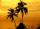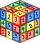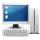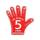# Geometric sequence 4

It is given geometric sequence a3 = 7 and a12 = 3. Calculate s23 (= sum of the first 23 members of the sequence).

Result

s23 =  83.26

#### Solution:Leave us a comment of example and its solution (i.e. if it is still somewhat unclear...):

Showing 0 comments:Be the first to comment!## Next similar examples:

1. Tenth memberCalculate the tenth member of geometric sequence when given: a1=1/2 and q=2
2. Geometric progression 2There is geometric sequence with a1=5.7 and quotient q=-2.5. Calculate a17.
3. Five membersWrite first 5 members geometric sequence and determine whether it is increasing or decreasing: a1 = 3 q = -2
4. Six termsFind the first six terms of the sequence a1 = -3, an = 2 * an-1
5. GP membersThe geometric sequence has 10 members. The last two members are 2 and -1. Which member is -1/16?
6. SequenceFind the common ratio of the sequence -3, -1.5, -0.75, -0.375, -0.1875. Ratio write as decimal number rounded to tenth.
7. BankPaul put 10000 in the bank for 6 years. Calculate how much you will have in the bank if he not pick earned interest or change deposit conditions. The annual interest rate is 3.5%, and the tax on interest is 10%.
8. GP - 8 itemsDetermine the first eight members of a geometric progression if a9=512, q=2
9. Eight palmThere grows 8 palms by the sea. At the first sitting one parrot, on second two, on third sits four parrots on each other twice the previous parrots sitting on a previous palm. How many parrots sitting on eighth palm?
10. A perineumA perineum string is 10% shorter than its original string. The first string is 24, what is the 9th string or term?
11. QuotientDetermine the quotient and the second member of the geometric progression where a3=10, a1+a2=-1,6 a1-a2=2,4.
12. ComputerThe computer was purchased 10000,-. Each year, the price of a computer depreciates always the same percentage of the previous year. After four years, the value of the computer is reduced to 1300,- How many percent was depreciated price of the computer each
13. Geometric progression 48,4√2,4,2√2
14. Median and modusRadka made 50 throws with a dice. The table saw fit individual dice's wall frequency: Wall Number: 1 2 3 4 5 6 frequency: 8 7 5 11 6 13 Calculate the modus and median of the wall numbers that Radka fell.
15. ValueFind the value of the expression: 6!·10^-3
16. Theorem proveWe want to prove the sentense: If the natural number n is divisible by six, then n is divisible by three. From what assumption we started?
17. Fish tankA fish tank at a pet store has 8 zebra fish. In how many different ways can George choose 2 zebra fish to buy?